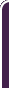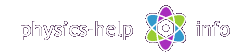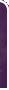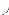homephysics online guidephysics help my info terms of use contactsWhat is Physics? Mechanics Electricity and Magnetism Electric Field Gauss' Law about below subjects Electric Potential Capacity Direct Current Magnetic Field Magnetic Field Laws Magnetic Interactions Electromagnetic Induction Maxwell's Equations Oscillations and Waves Simple Harmonic Motion Damped Harmonic Motion Driven Harmonic Motion Electric Oscillation Alternating Current Wave Motion Elastic Waves Electromagnetic Waves Optics Light Waves Geometrical Optics Interference Polarization Diffraction Fraunhofer Diffraction Dispersion, Absorption, Diffusion Doppler Effect Thermodynamics Ideal Gas Molecular Statistics Transport Phenomena First Law of Thermodynamics Second and Third Laws of Thermodynamics Imperfect Gas Liquids Solids Quantum Physics Thermal Radiation Quantum Properties of Light Wave Properties of Particles Planetary Model of Atom X-Rays Particle in Potential Well Pauli Exclusion Principle Nuclear Physics Solid State Physics Appendices# Rotational Dynamics

SI units & Physics constants

Rotational dynamics investigates rotational motion of objects and deals with effects that forces have on motion

## Rotation of point particleHere (all units see here):

m is mass of the particle moving in x-y planeis force vector applied in the plane of motionis velocity vector, tangent to trajectoryis linear momentum vector, parallel tois radius vector of curvature of trajectory, normal to trajectoryis angular velocity vector, normal to plane of motionis angular acceleration vector, normal to plane of motionis angular momentum, parallel tois torque associated with the force, normal to plane of motion

d is level arm of#### General formulas

Moment of inertia of the particle about center of rotationAngular momentum vector is defined by vector productwhereis linear momentum vector, perpendicular toRelation between angular momentum and angular velocity vectorsThe magnitude of angular momentumTorque vector is defined by vector productThe magnitude of torquewhere:is angle between vectorsand, shown in the above diagramis level arm (or moment arm) ofNewton's Second Law in angular form:

- for general case- for constant moment of inertia## Plane rotation of symmetric solid about its axis of symmetry

Moment of inertia of the solid about axis of rotationwhere

mi is small portion of mass number i at distance Ri between its center and axis of rotation (for i = 1, 2, 3, ... , n)

dV is infinitesimal volume with densityat distance R from axis of rotation

Parallel Axis Theoremwhere:

I is moment of inertia of solid of mass m about axis located at distance l from its center of mass

Icm is moment of inertia of the solid about axis passing thought the ceneter of mass and parallel the the previous axis

Angular momentumwhereis angular velocity of the solid

Newton's Second Law in angular form:

- for general case- for constant moment of inertiawhereis net torque about axis of rotation associated with net external force

## General case for rotation of system of particles

Resultant angular momentum vector of the system about arbitrary point Cwhereandare position vector and linear momentum vector for i-th particle with respect to the point C (for  i = 1, 2, 3, ..., n)

Resultant torque about point C associated with external forceswhereis external force applied at pointwith respect to the point C (for j = 1, 2, 3, ..., k)

Newton's Second Law in angular formLaw of conservation of angular momentum of the system

Ifthenabout point C

## Gyroscopic motion of spinning topHere:is angular velocity of the top about its axisis vertical external force applied to the topis radius-vector of the point where the forceis applied to the topis precessional frequency of the top about z-axis

Equation of motion for the topwhere I is moment of inertia of the top about it's axis

The value of precessional frequencyhome  |  physics online guide  |  physics help  |  my info  |  terms of use  |  contacts Dr. Nikitin © 2010